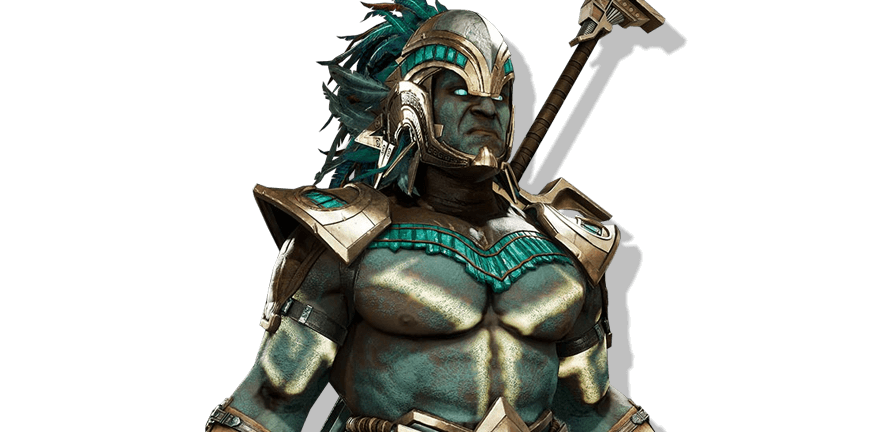Start learning movesets and combos!

Choose the platform you play on.
See how to read and perform inputs.Mortal Kombat 11

# Kotal KahnCombos

Kotal native name is Ko'atal. His Khan title was inherited after defeating Shao Khan.Know more

Get notified of the character’s updates

## Basic combo attacks

Combinations of basic moves

One
Two

Two
Two

Three
Four

One
Two
Two

Forward
+
One
Two

Forward
+
One
Two
Two

Forward
+
One
Four

Two
Two
One

Forward
+
Two
Four

Forward
+
Two
Four
Three

Back
+
Two
Two

Back
+
Two
Two
Three

### Melahuac

Forward
+
Three
Four

Long combinations of various moves including abilities and specials

Two
Two
One
Forward
Forward
Forward
+
One
Two
Two

Back
+
Two
Two
Down
Forward
Two
Three
Four
Down
Forward
Two

Forward
+
Two
Four
Down
Forward
Two
Forward
Forward
Down
+
One
Forward
+
One
Two
Two

Forward
+
One
Two
Two
KB
Forward
Forward
Three
Four
Back
Forward
Four

Two
Two
One
Forward
Forward
Forward
+
One
Two
Back
Forward
Four

Forward
+
Two
Four
Back
Forward
Four

Two
Two
One
Forward
Forward
Forward
+
One
Two
Down
Forward
Two

Back
+
Two
Two
Forward
Forward
Three
Four
Down
Forward
Two

Down
+
Two
KB
Forward
Forward
Three
Four
Down
Forward
Two